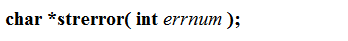# C语言中那些“不受限制”的字符串函数

## “不受限制的“字符串函数

### strlen

strlen函数用于求解字符串长度，其返回类型为unsigned int（即size_t）。strlen函数从起点开始，往后计数，遇到‘\0’停止。

``````#include<string.h>
#include<iostream>
using namespace std;

int main()
{
if ((strlen("abc") - strlen("abcdef")) > 0)
cout << "大于" << endl;
else
cout << "小于" << endl;

return 0;
}
``````

### strcpy

strcpy函数是字符串拷贝函数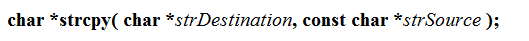``````#include<string>
#include<iostream>
using namespace std;
int main()
{
char arr = "abcdefgh******";
char* p = "hello world";
strcpy(arr, p);
cout << arr << endl;
return 0;
}
``````

### strcmp

strcmp函数为字符串比较函数。比较的不是字符串的长度，而是对应字符的ASCII值。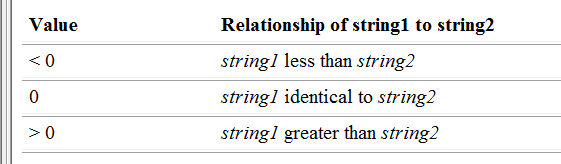``````#include<string>
#include<iostream>
using namespace std;
int main()
{
char arr[] = "abcde";
char p[] = "abde";
cout << strcmp(arr,p) << endl;

return 0;
}
``````

### strcat

strcat函数为字符串追加函数。函数原型：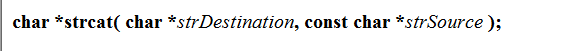``````int main()
{
char arr = "abcde ";
char p[] = "hello";
cout << strcat(arr, p) << endl;

return 0;
}
``````

### strstr

strstr为字符串查找函数。函数原型为：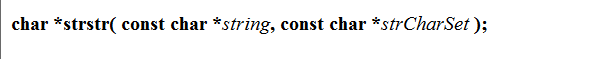``````int main()
{
char arr[] = "hello, how are you ?";
char p[] = "are";
cout << strstr(arr, p) << endl;

return 0;
}
``````

### strtok

strtok函数为字符串分隔函数。函数原型为：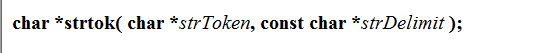strDelimit为字符串，定义了分隔字符的集合。函数执行是遇到分割字符时，将它改为‘\0’，范围一个指向这个标记的指针。注意：strtok函数执行时修改了字符串的内容。

``````int main()
{
char str[] = "hello,how are you ?";
char * p;
p = strtok(str, ",?");
while (p != NULL)
{
printf("%s\n", p);
p = strtok(NULL, ",?");
}
return 0;
}
``````

### strerror

strerror函数的作用：返回错误码对应的信息。函数原型为